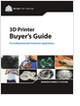MaterialsDesign CenterProcessesUnit ConversionFormulasMathematicsCalculatorsDiscussion ForumTrade PublicationsDirectory Service
Essentials of Manufacturing

Information, coverage of important developments and expert commentary in manufacturing.

Selecting the Right 3D Printer

Discover how to choose the right 3D printer for your needs and the key performance attributes to consider.

Metal 3D Printing Design Guide

Direct Metal Laser Sintering (DMLS) 3D printing for parts with reduced cost and little waste.

Mechanical Engineers Outlook

Guide for those interested in becoming a mechanical engineer. Includes qualifications, pay, and job duties.

more free publicationsGlossary » Units » Pressure » Micron of Mercury (millitorr)Micron of Mercury (millitorr) (µHg (0 °C)) is a unit in the category of Pressure. It is also known as microns of mercury, micron of hg, microns of hg. Micron of Mercury (millitorr) (µHg (0 °C)) has a dimension of ML-1T-2 where M is mass, L is length, and T is time. It can be converted to the corresponding standard SI unit Pa by multiplying its value by a factor of 0.1333223684.
Note that the seven base dimensions are M (Mass), L (Length), T (Time), Q (Temperature), N (Aamount of Substance), I (Electric Current), and J (Luminous Intensity).

Other units in the category of Pressure include Atmosphere (metric) (at), Atmosphere (standard) (atm), Bar (bar), Barad (barad), Barye, CentiHg (0°C), Centimeter of Mercury (0°C) (cmHg (0 °C)), Centimeter of Water (4°C) (cmH2O), Dyne Per Square Centimeter (dyn/cm2), Foot of Water (4°C) (ft H2O), Gigapascal (GPa), Hectopascal (hPa), Inch of Mercury (0°C) (inHg (0 °C)), Inch of Mercury (15.56°C) (inHg (15.56 °C)), Inch of Water (15.56°C) (inH2O (15.56 °C)), Inch of Water (4°C) (inH2O (4 °C)), Kilogram Force Per Square Centimeter (kgf/cm2), Kilogram Force Per Square Decimeter (kgf/dm2), Kilogram Force Per Square Meter (kgf/m2), Kilogram Force Per Square Millimeter (kgf/mm2), Kilopascal (kPa), Kilopound Force Per Square Inch (kip/in2, ksi, KSI), Megapascal (MPa), Meter of Water (15.56°C) (mH2O, mCE (15.56 °C)), Meter of Water (4°C) (mH2O, mCE (4 °C)), Microbar (barye, Barrie) (µbar), Millibar (mbar), Millimeter of Mercury (0°C) (mmHg, torr, Torr (0 °C)), Millimeter of Water (15.56°C) (mmH2O, mmCE (15.56 °C)), Millimeter of Water (4°C) (mmH2O, mmCE (4 °C)), Millitorr (mtorr), Newton Per Square Meter (N/m2), Ounce Force (av.) Per Square Inch (ozf/in2, osi), Pascal (Pa, N/m2), Pound Force Per Square Foot (lbf/ft2), Pound Force Per Square Inch (psi, PSI, lbf/in2), Poundal Per Square Foot (pdl/ft2), Poundal Per Square Inch (pdl/in2), Standard Atmosphere (atm), Ton Force (long) Per Square Foot (tonf/ft2 (UK)), Ton Force (long) Per Square Inch (tonf/in2 (UK)), Ton Force (metric) Per Square Centimeter (tonf/cm2 (metric)), Ton Force (metric) Per Square Meter (tonf/m2 (metric)), Ton Force (short) Per Square Foot (tonf/ft2 (US)), Ton Force (short) Per Square Inch (tonf/in2 (US)), and Torr (torr).N/ARelated PagesGlossaryEssentials of Manufacturing

Information, coverage of important developments and expert commentary in manufacturing.Selecting the Right 3D Printer

Discover how to choose the right 3D printer for your needs and the key performance attributes to consider.Metal 3D Printing Design Guide

Direct Metal Laser Sintering (DMLS) 3D printing for parts with reduced cost and little waste.Mechanical Engineers Outlook

Guide for those interested in becoming a mechanical engineer. Includes qualifications, pay, and job duties.